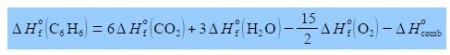# Enthalpy of Combustion of Benzene

When benzene, below Figure, is combusted it has the following stoichiometric reaction

C6 H6 + 15/2 O2 → 6CO2 + 3 H2O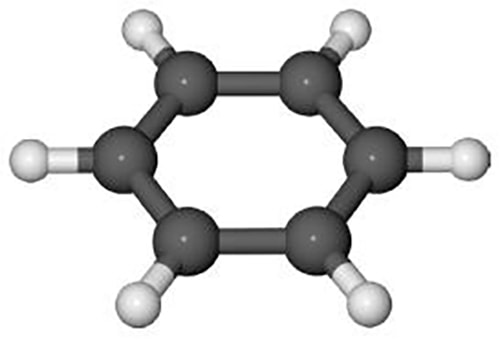The enthalpy change for this combustion reaction is equal to the sum of the standard enthalpies of formation ΔH0 of the products minus the sum of the standard enthalpies of formation of the reactants. Each enthalpy of the formation being multiplied by the stoichiometric coefficient for that molecule. Enthalpy of formation is covered during the first year of your degree.Rearrange the enthalpy equation to get the enthalpy of combustion of benzene as the subject of the equation.

Solution

In order to not get lost in the algebra: (1) expand out the bracket on the right-hand side;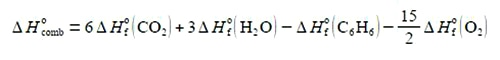(2) take ΔH0 (C6 H6) over to the left-hand side by adding ΔH0 (C6 H6)  to both sides of the equation and cancelling out where possible;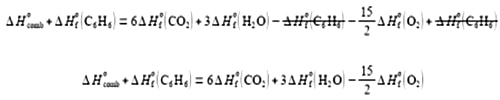(3) take the enthalpy of combustion ΔH0comb to the right-hand side by subtracting ΔH0comb from both sides and then cancelling out where possible.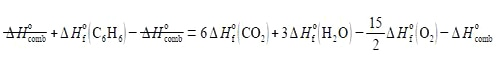(4) which then gives the heat of formation of combustion of benzene as the subject of the equation.